# Recursion To understand recursion one must first understand

• Slides: 42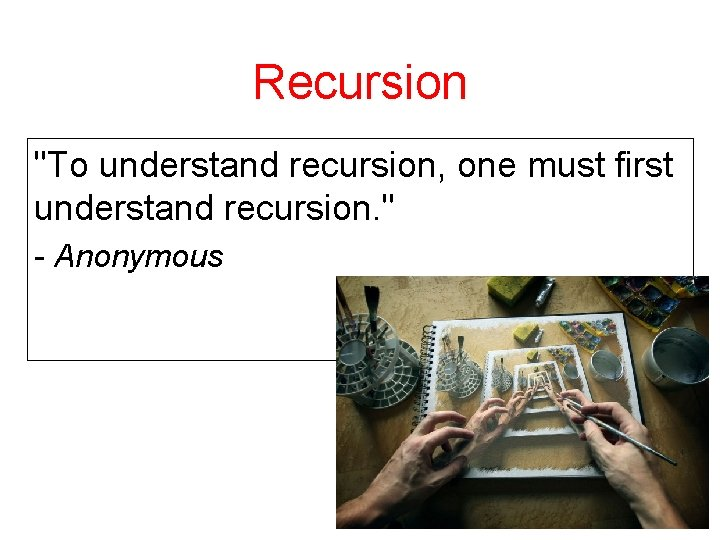Recursion "To understand recursion, one must first understand recursion. " - Anonymous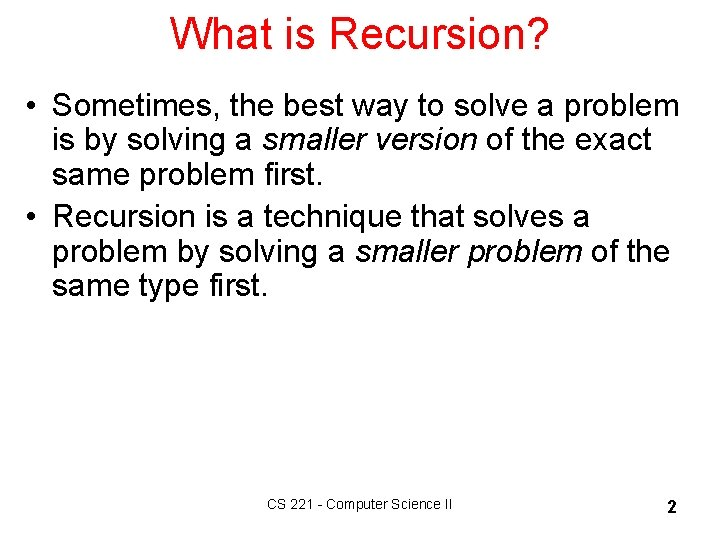What is Recursion? • Sometimes, the best way to solve a problem is by solving a smaller version of the exact same problem first. • Recursion is a technique that solves a problem by solving a smaller problem of the same type first. CS 221 - Computer Science II 2Recursion • More than programming technique: • A way of describing, defining, or specifying things. • A way of designing solutions to problems (divide and conquer). CS 221 - Computer Science II 3Basic Recursion 1. Base cases: – Always have at least one case that can be solved without using recursion. – Indicates an end to the recursion. 2. Make progress: – Any recursive call must make progress toward a base case. CS 221 - Computer Science II 4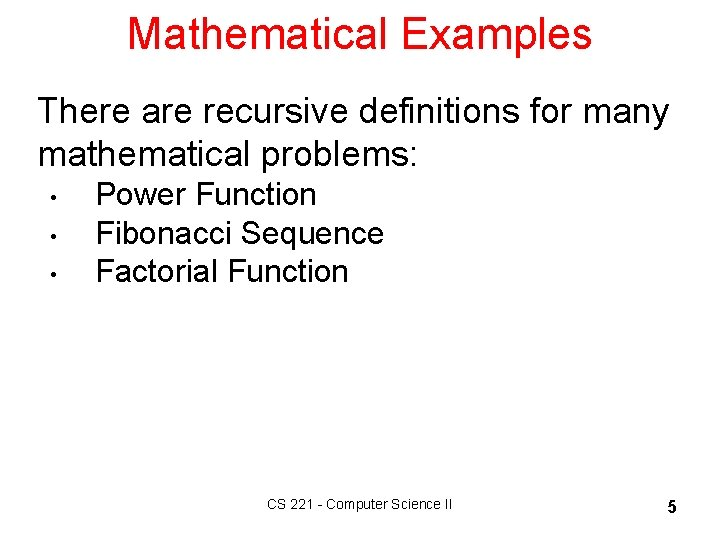Mathematical Examples There are recursive definitions for many mathematical problems: • • • Power Function Fibonacci Sequence Factorial Function CS 221 - Computer Science II 5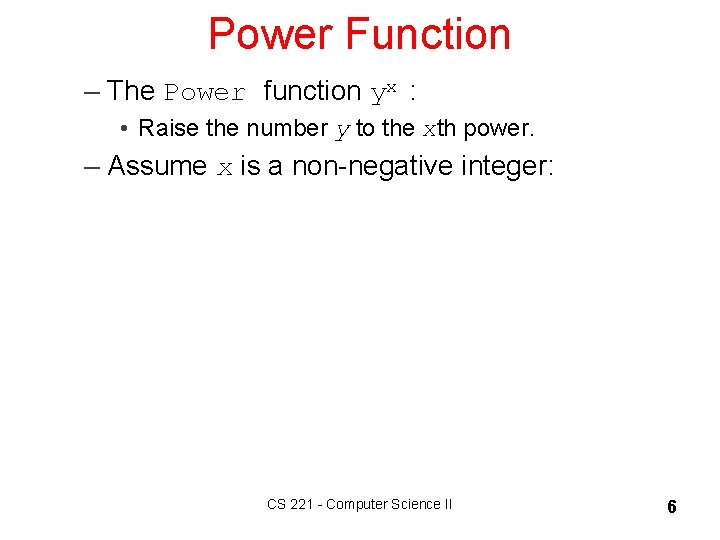Power Function – The Power function yx : • Raise the number y to the xth power. – Assume x is a non-negative integer: CS 221 - Computer Science II 6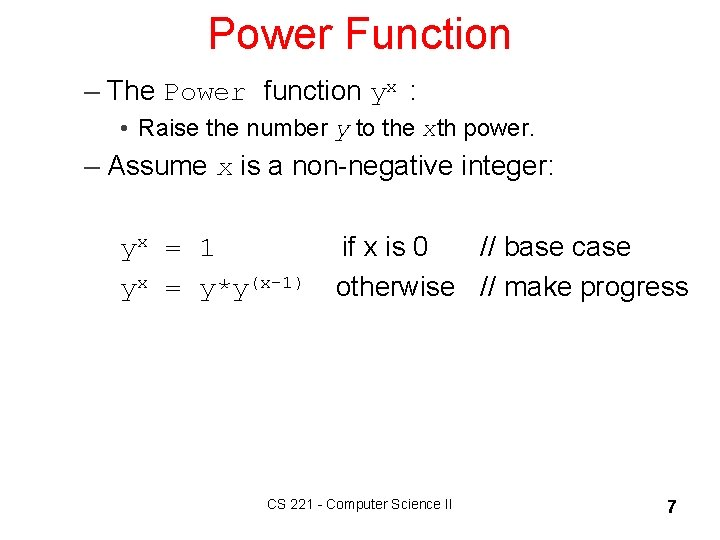Power Function – The Power function yx : • Raise the number y to the xth power. – Assume x is a non-negative integer: yx = 1 if x is 0 // base case yx = y*y(x-1) otherwise // make progress CS 221 - Computer Science II 7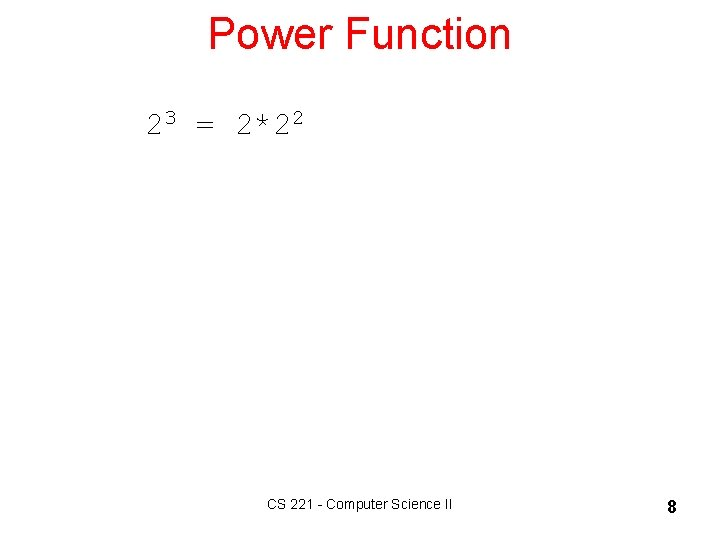Power Function 23 = 2*22 CS 221 - Computer Science II 8Power Function 23 = 2 * 22 22 = CS 221 - Computer Science II 9Power Function 23 = 2 * 22 22 = 2 * 21 = CS 221 - Computer Science II 10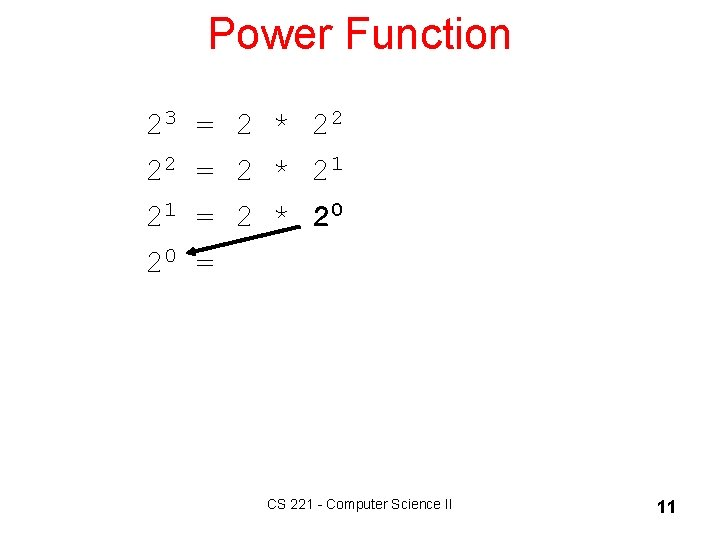Power Function 23 = 2 * 22 22 = 2 * 21 = 2 * 20 = CS 221 - Computer Science II 11Power Function 23 = 2 * 22 22 = 2 * 21 = 2 * 20 = 1 (Base Case) CS 221 - Computer Science II 12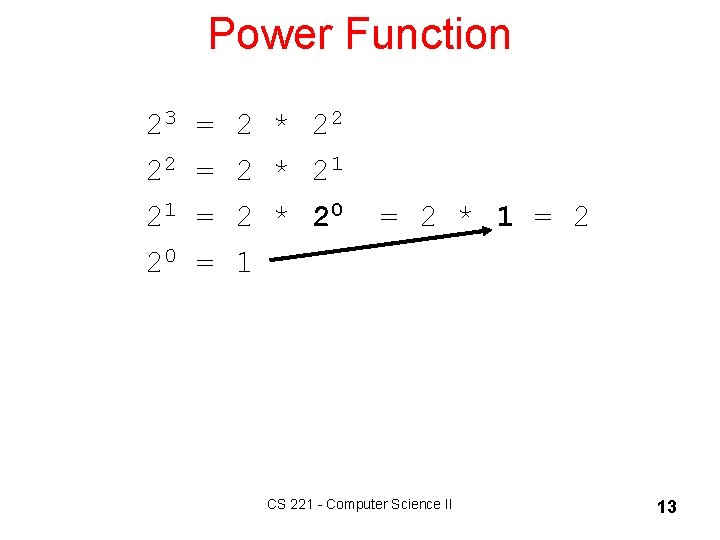Power Function 23 = 2 * 22 22 = 2 * 21 = 2 * 20 = 2 * 1 = 2 20 = 1 CS 221 - Computer Science II 13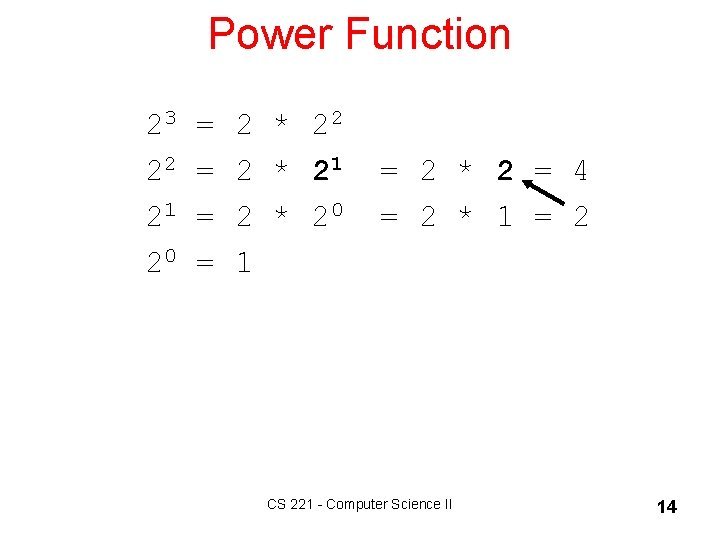Power Function 23 = 2 * 22 22 = 2 * 21 = 2 * 2 = 4 21 = 2 * 20 = 2 * 1 = 2 20 = 1 CS 221 - Computer Science II 14Power Function 23 = 2 * 22 = 2 * 4 = 8 22 = 2 * 21 = 2 * 2 = 4 21 = 2 * 20 = 2 * 1 = 2 20 = 1 CS 221 - Computer Science II 15Fibonacci Sequence: 1, 1, 2, 3, 5, 8, 13, 21, 34, 55, 89, 144, … Fibonacci Function: Fib(0) = 1 // base case Fib(1) = 1 // base case Fib(n) = Fib(n-1) + Fib(n-2) // n>1 Unlike most recursive algorithms: § Two base cases, not just one. § Two recursive calls, not just one. CS 221 - Computer Science II 16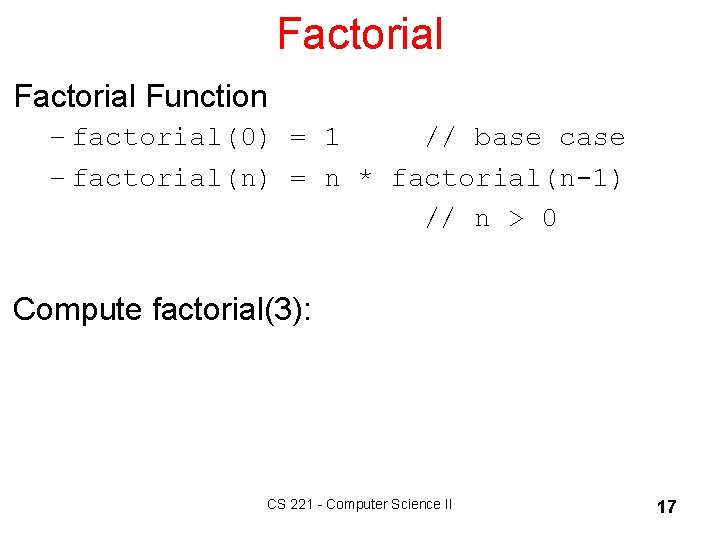Factorial Function – factorial(0) = 1 // base case – factorial(n) = n * factorial(n-1) // n > 0 Compute factorial(3): CS 221 - Computer Science II 17Factorial Function – factorial(0) = 1 // base case – factorial(n) = n * factorial(n-1) // n > 0 Compute factorial(3): factorial(3) = 3 * factorial(2) = 3 * ( 2 * factorial(1) ) = 3 * ( 2 * ( 1 * factorial(0) ) = 3 * ( 2 * ( 1 * 1 ) )) = 6 CS 221 - Computer Science II 18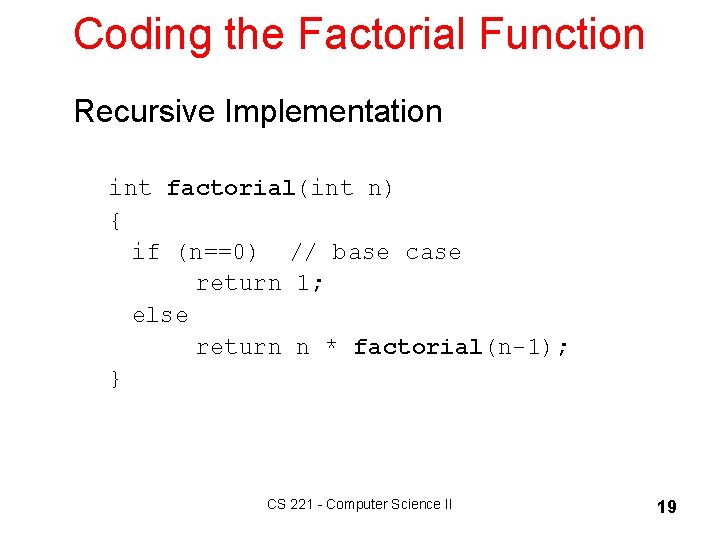Coding the Factorial Function Recursive Implementation int factorial(int n) { if (n==0) // base case return 1; else return n * factorial(n-1); } CS 221 - Computer Science II 19Recursive Call Stack CS 221 - Computer Science II 20Implementing Recursion What happens when a function gets called? // a method int b(int x) { int z, y; // other statements z = a(x) + y; return z; } // another method int a(int w) { return w+w; } CS 221 - Computer Science II 21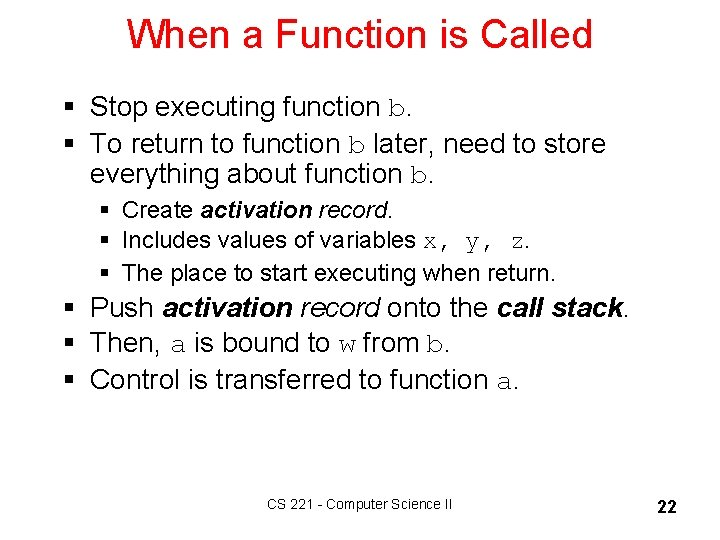When a Function is Called § Stop executing function b. § To return to function b later, need to store everything about function b. § Create activation record. § Includes values of variables x, y, z. § The place to start executing when return. § Push activation record onto the call stack. § Then, a is bound to w from b. § Control is transferred to function a. CS 221 - Computer Science II 22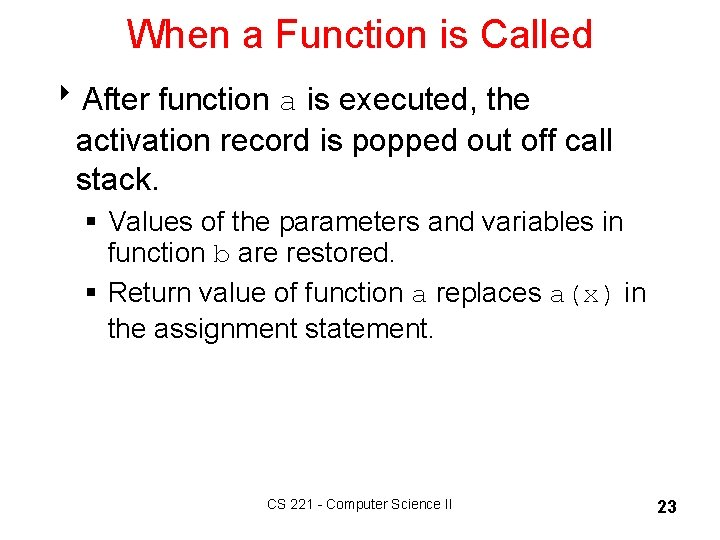When a Function is Called 8 After function a is executed, the activation record is popped out off call stack. § Values of the parameters and variables in function b are restored. § Return value of function a replaces a(x) in the assignment statement. CS 221 - Computer Science II 23Recursion Overhead § Space § Every invocation of a function call requires: § Space for parameters and local variables. § Space for return address. § Thus, a recursive algorithm needs space proportional to the number of nested calls to the same function. CS 221 - Computer Science II 24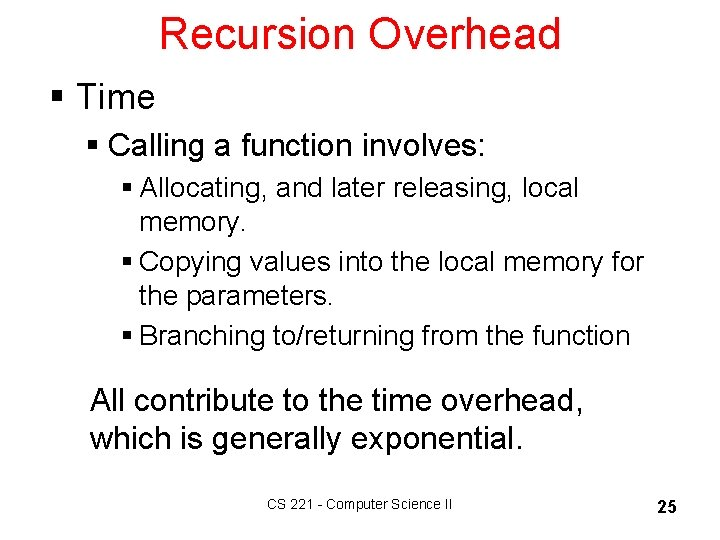Recursion Overhead § Time § Calling a function involves: § Allocating, and later releasing, local memory. § Copying values into the local memory for the parameters. § Branching to/returning from the function All contribute to the time overhead, which is generally exponential. CS 221 - Computer Science II 25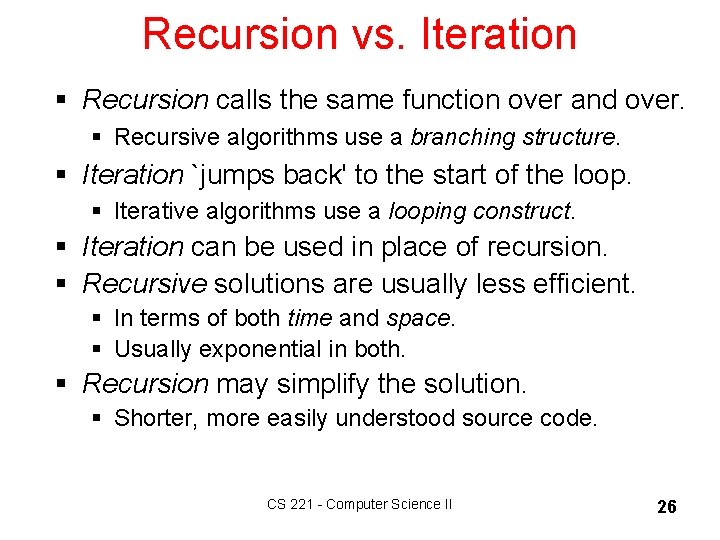Recursion vs. Iteration § Recursion calls the same function over and over. § Recursive algorithms use a branching structure. § Iteration `jumps back' to the start of the loop. § Iterative algorithms use a looping construct. § Iteration can be used in place of recursion. § Recursive solutions are usually less efficient. § In terms of both time and space. § Usually exponential in both. § Recursion may simplify the solution. § Shorter, more easily understood source code. CS 221 - Computer Science II 26Coding Factorial Function Iterative implementation: int factorial(int n) { int fact = 1; for(int count = 2; count <= n; count++) fact = fact * count; return fact; } CS 221 - Computer Science II 27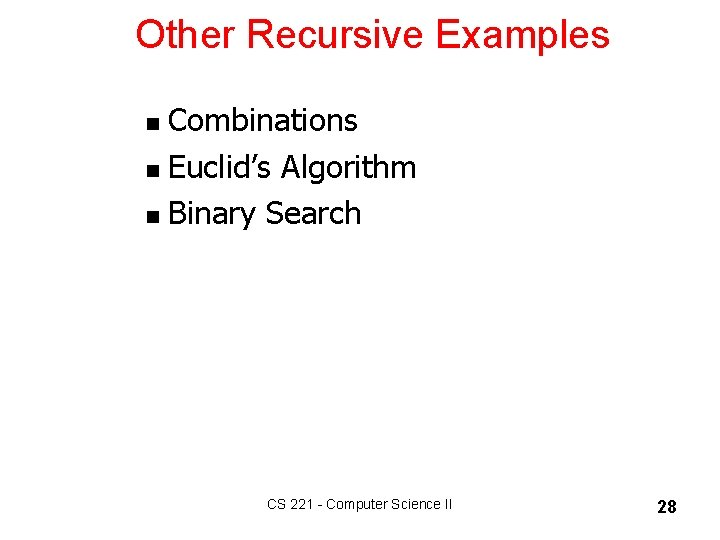Other Recursive Examples Combinations n Euclid’s Algorithm n Binary Search n CS 221 - Computer Science II 28Combinations: n choose k Given n things, how many different sets of size k can be chosen? n n-1 = + k-1 , 1 < k < n (recursive solution) k k n n! = , 1 < k < n (closed-form solution) k k!(n-k)! with base cases: n n = n (k = 1), = 1 (k = n) 1 n Pascal’s Triangle CS 221 - Computer Science II 29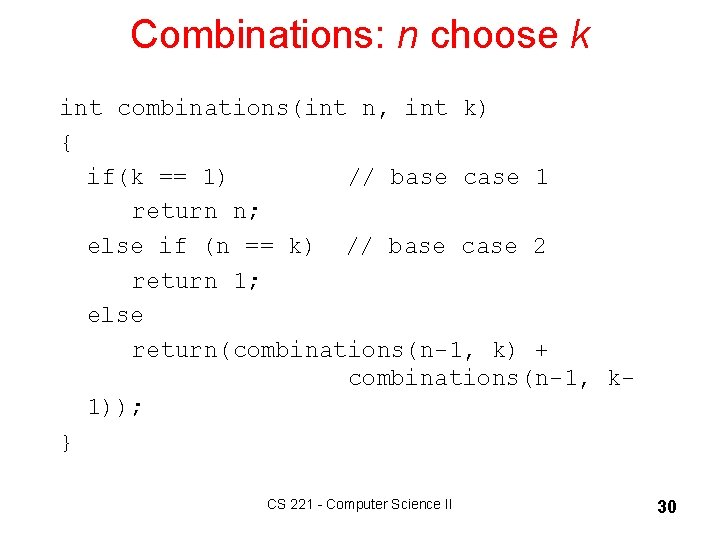Combinations: n choose k int combinations(int n, int k) { if(k == 1) // base case 1 return n; else if (n == k) // base case 2 return 1; else return(combinations(n-1, k) + combinations(n-1, k 1)); } CS 221 - Computer Science II 30Combinations 31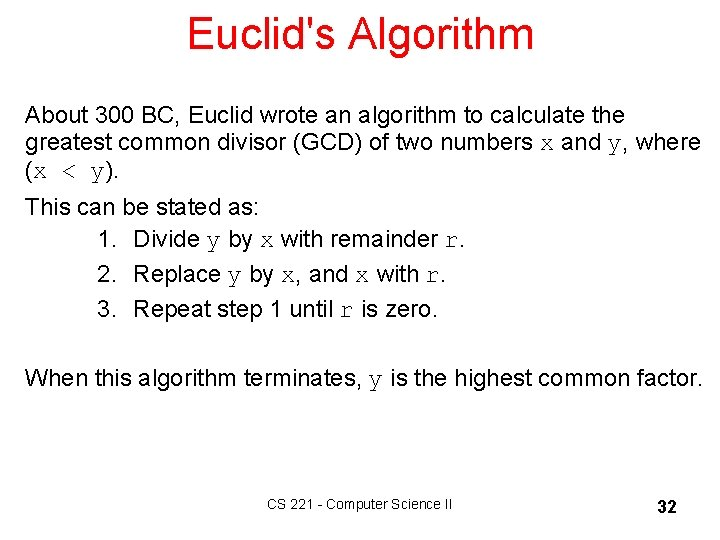Euclid's Algorithm About 300 BC, Euclid wrote an algorithm to calculate the greatest common divisor (GCD) of two numbers x and y, where (x < y). This can be stated as: 1. Divide y by x with remainder r. 2. Replace y by x, and x with r. 3. Repeat step 1 until r is zero. When this algorithm terminates, y is the highest common factor. CS 221 - Computer Science II 32GCD(34017, 16966) Euclid's algorithm works as follows: – 34, 017/16, 966 produces a remainder 85 – – 16, 966/85 produces a remainder 51 85/51 produces a remainder 34 51/34 produces a remainder 17 34/17 produces a remainder 0 The highest common divisor of 34, 017 and 16, 966 is 17. CS 221 - Computer Science II 33Writing a Recursive Function 1. Determine the base case(s). – Can solve without recursion. 2. Determine the general case(s). – Express solution as a smaller version of itself. 3. Verify the algorithm. – Use the "Three-Question-Method. " CS 221 - Computer Science II 34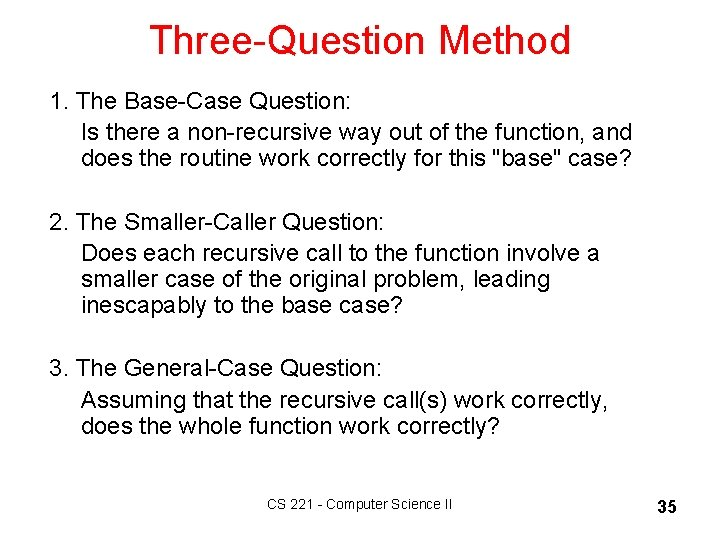Three-Question Method 1. The Base-Case Question: Is there a non-recursive way out of the function, and does the routine work correctly for this "base" case? 2. The Smaller-Caller Question: Does each recursive call to the function involve a smaller case of the original problem, leading inescapably to the base case? 3. The General-Case Question: Assuming that the recursive call(s) work correctly, does the whole function work correctly? CS 221 - Computer Science II 35Binary Search § Search algorithm § Finds a target value within a sorted list. § Compares target value to the middle element. § If the two are equal, done. § If target less than middle element, search lower half of list. Otherwise, search upper half of list. § Continue dividing list in half until find target or run out of list to search. § Efficiency: § Runs in at worst logarithmic O(log n) time. § Takes up linear O(n) space. CS 221 - Computer Science II 36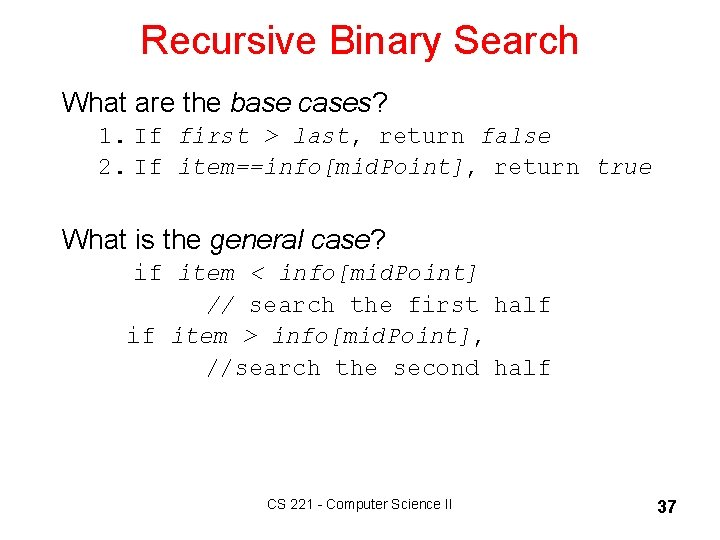Recursive Binary Search What are the base cases? 1. If first > last, return false 2. If item==info[mid. Point], return true What is the general case? if item < info[mid. Point] // search the first half if item > info[mid. Point], //search the second half CS 221 - Computer Science II 37Recursive Binary Search boolean binary. Search(Item info[], Item item, int first, int last) { int mid. Point; if(first > last) // base case 1 return false; else { mid. Point = (first + last)/2; if (item == info[mid. Point]) // base case 2 { item = info[mid. Point]; return true; } else if(item < info[mid. Point]) return binary. Search(info, item, first, mid. Point-1); else return binary. Search(info, item, mid. Point+1, last); } } CS 221 - Computer Science II 38When to Use Recursion § When the depth of recursive calls is relatively "shallow. " § The recursive version does about the same amount of work as the nonrecursive version. § The recursive version is shorter and simpler than the non-recursive solution. CS 221 - Computer Science II 39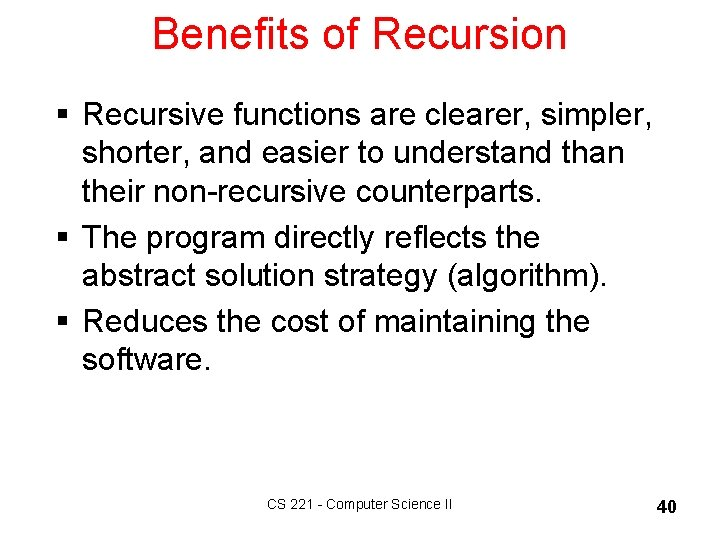Benefits of Recursion § Recursive functions are clearer, simpler, shorter, and easier to understand than their non-recursive counterparts. § The program directly reflects the abstract solution strategy (algorithm). § Reduces the cost of maintaining the software. CS 221 - Computer Science II 40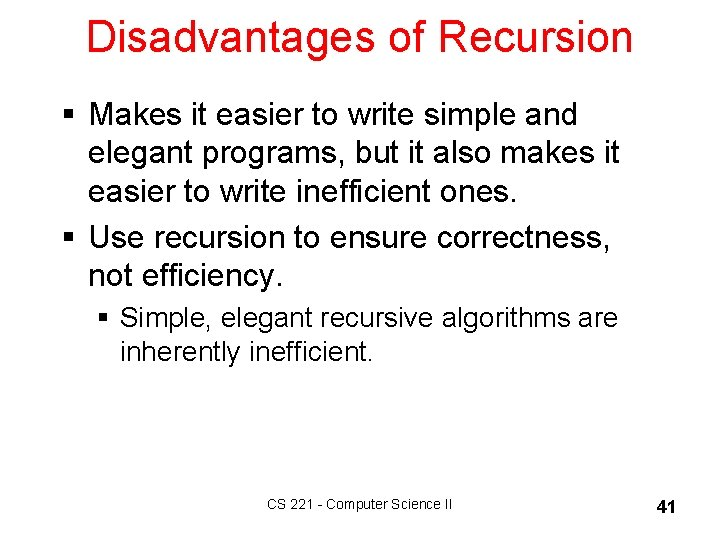Disadvantages of Recursion § Makes it easier to write simple and elegant programs, but it also makes it easier to write inefficient ones. § Use recursion to ensure correctness, not efficiency. § Simple, elegant recursive algorithms are inherently inefficient. CS 221 - Computer Science II 41CS 221 - Computer Science II 42